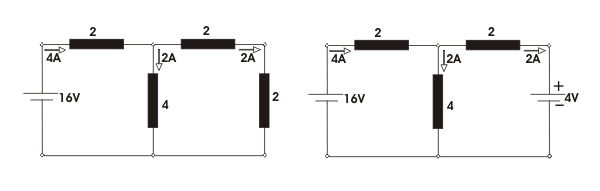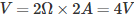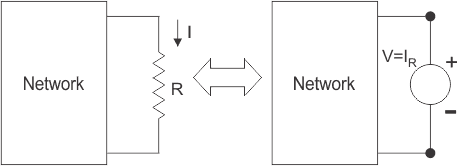# Compensation Theorem

## Concept of Compensation Theorem

This theorem is based on one basic concept. According to Ohm’s law, when current flows through any resistor, there would be a voltage drop across the resistor. This dropped voltage opposes the source voltage. Hence voltage drop across a resistance in any network can be assumed as a voltage source acting opposite to the source voltage. The compensation theorem depends upon this concept.

## Explanation of Compensation Theorem

According to this theorem, any resistance in a network may be replaced by a voltage source that has zero internal resistance and a voltage equal to the voltage drop across the replace resistance due to the current which was flowing through it.

This imaginary voltage source is directed opposite to the voltage source of that replaced resistance. Think about a resistive branch of any complex network that’s value is R. Let’s assume current I is flowing through that resistor R and voltage drops due to this current across the resistor is V = I.R. According to compensation theorem, this resistor can be replaced by a voltage source that’s generated voltage will be V (= IR) and will be directed against the direction of network voltage or direction of current I.

## Example of Compensation Theorem

The compensation theorem can easily be understood by this following example.Here in the network for 16V source, all the currents flowing through the different resistive branches are shown in the first figure. The current through the right most branch in the figure is 2A and its resistance is 2 Ω. If this right most branch of the network is replaced by a voltage sourcedirected as shown in the second figure, then current through the other branches of the network will remain the same as shown in the second figure.Want To Learn Faster? 🎓
Get electrical articles delivered to your inbox every week.
No credit card required—it’s 100% free.